## Monday, March 28, 2016

### Multisort in jqGrid does not work like excel

The idea of multisort is that when a grid is sorted by one column and then by another, the first sort must stay in place, and yet the second column must sort dependently of the first.
Example:
See the below grid
Pic1
 digit char tex 1 a xyz 1 b mno 2 a abc 2 b xyz 2 c lmn 3 a abc

This grid is sorted by the column ‘digit’ – as you can see all the values in the digit column are ascending.
If we sort the column ‘char’ then we get the below result
Pic2
 digit char tex 1 a xyz 2 a abc 3 a abc 1 b mno 2 b xyz 2 c lmn

Now if we sort by the digit column in ascending order, the above grid should remain unchanged – notice that first sort column (char) is already sorted and in a multisort, we need to sort the second (digit) column within the sorted bounds of the first sort column. Therefore, the resulting grid (upon sorting the digit column) is the same one.
This is how excel sorts the data. Look at the screenshot below

Pic3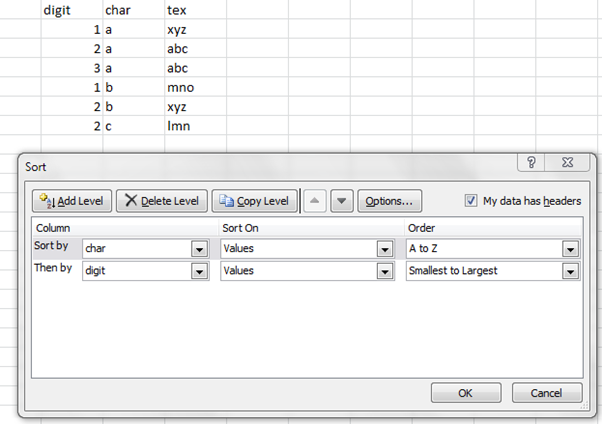Above image shows an excel page with the same grid on which custom sort is used to generate the result.

Now how is this different from the multisort function of the jqGrid?
For this I have created the exact same grid locally to perform the same operations. Initially the grid looks like:
Pic4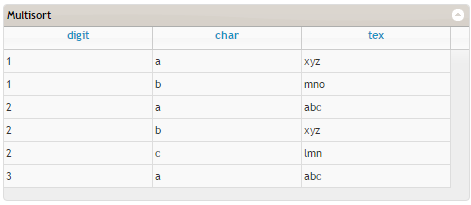Notice that none of the columns have been sorted.

If we sort the grid by clicking on the ‘char’ column, we the following result
Pic5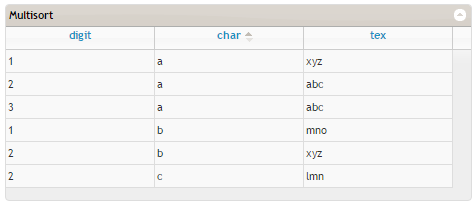This is the same result obtained with excel (see Pic2)
Till here we have common sorting
Now, if we sort by the ‘digit’ column, the sorted data on ‘char’ column does not remain in place
the second column is sorted independently of the first. See the result

Pic6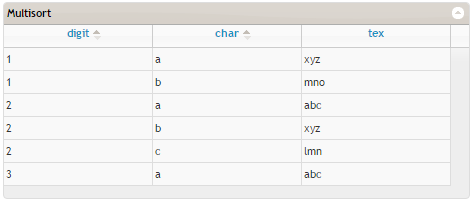You can see that the digit column has been sorted independently of the ‘char’ column. As per the excel results, the ‘digit’ column should have sorted internally according to the sort in the char column.
See both the results
Pic7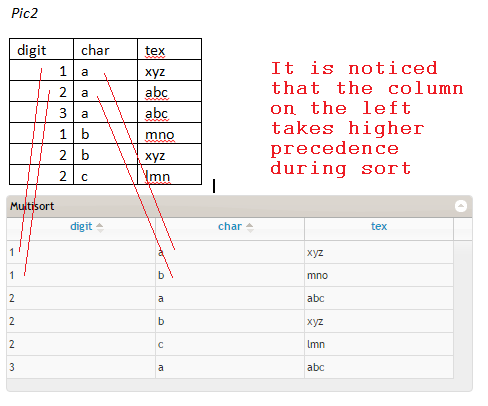See the picture above that shows both results. (Also take note of the note in the image above)
The jqGrid sorts fine when the sort columns are selected from left to right
I noticed that when the sort order is chosen from left to right, the multisort works fine.
Example if the column tex is chosen instead of ‘digit’, the result grid after the first sort (ie sort by ‘char’) will be
Pic8
 digit char tex 2 a abc 3 a abc 1 a xyz 1 b mno 2 b xyz 2 c lmn

Below is the complete scenario
Pic9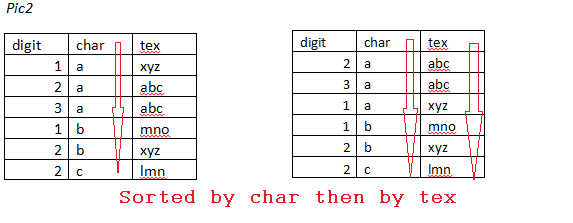As per excel:

Pic10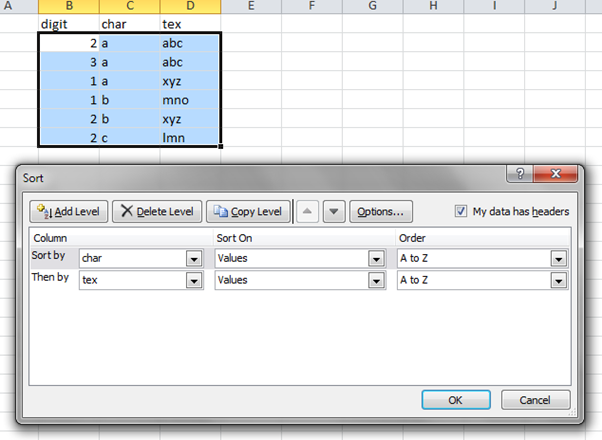When this same scenario is used in a jqGrid, the following result is obtained
Pic11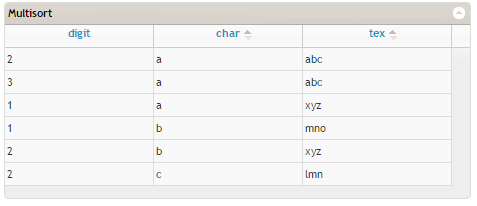Below is the combined screen explained:
Pic12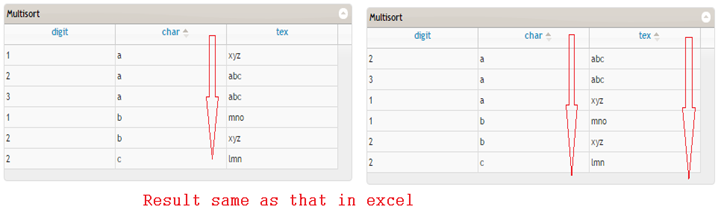Above shows that the jqGrid results match with those got from excel.

Conclusion:
The multisort feature of the jqGrid works will from left to right i.e. It works well if the columns are sorted by that order, if sorted by clicking on columns not is order then the sort fails.
The technical reasoning can be given from the JavaScript of the plugin itself. When sorted columns are retrieved via the jqGrid function called jqGrid('getGridParam','sortname');
that retrieves the column names from left to right.

The code used to generate the jqGrid is given below
<!DOCTYPE html PUBLIC "-//W3C//DTD XHTML 1.0 Transitional//EN" "http://www.w3.org/TR/xhtml1/DTD/xhtml1-transitional.dtd">
<html xmlns="http://www.w3.org/1999/xhtml">
<title>Multisort test</title>
<script src="http://trirand.com/blog/jqgrid/js/jquery.jqGrid.min.js" type="text/javascript"></script>
<script src="http://trirand.com/blog/jqgrid/js/i18n/grid.locale-en.js" type="text/javascript"></script>
<script type="text/javascript">
var myData = [{ digit: 1, char: "a", tex: "xyz" }, { digit: 1, char: "b", tex: "mno" }, { digit: 2, char: "a", tex: "abc" }, { digit: 2, char: "b", tex: "xyz" }, { digit: 2, char: "c", tex: "lmn" }, { digit: 3, char: "a", tex: "abc"}];
\$("#grid").jqGrid({
datatype: "local",
data:myData,
colNames: ['digit', 'char', 'tex'],
colModel: [{ name: 'digit' }, { name: 'char'}, { name: 'tex'}],
caption: "Multisort",
multiSort: true
});
});

</script>